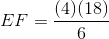## Example Questions

### Example Question #1 : How To Find The Length Of The Side Of A Trapezoid

Given the height of a trapezoid isand a base length is, what is the length of the other base if the area of the trapezoid is?Explanation:

Write the formula used to find the area of a trapezoid.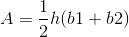Substitute the given information to the formula and solve for the unknown base.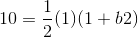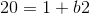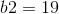### Example Question #2 : How To Find The Length Of The Side Of A Trapezoid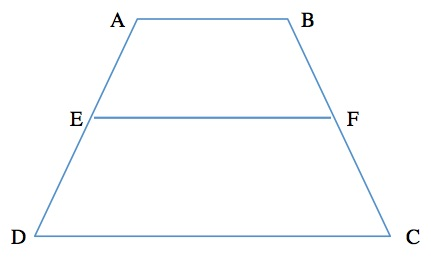is an isosceles trapezoid that is bisected by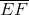.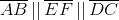. If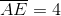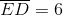, and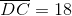, then what is the length of?Explanation:

We know that all three horizontal lines are parallel to one another. By definition, we can set up a ratio between the lengths of the sides provided to us in the question and the lengths of the two parallel lines: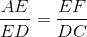Once we substitute the given information, we get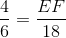We cross multiply to solve for EF